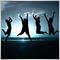• Visão global
• Comentários (1)
• Discussão (2)
• O que há de novo

# Martingale Classic

Martingale Vortex is a system of classic martingale trading supplemented with the ability to affect the main parameters depending on the depth.

• Does not use indicators;
• Works on both flat and trending markets;
• Not afraid of the gaps;
• Uses virtual StopLoss and TakeProfit levels;
• Automatically detects suffixes and prefixes of the traded instruments;
• Works with 5-digit and 4-digit quotes;
• Protection from deposit wipe-out.

### Input Parameters

• CloseAll - close all open orders, true - close, also disables trading (even if Trade = true);
• Magic - EA magic number;
• Lots - volume of the first order;
• Step - the distance in points by the 4th digit, when reached the next order with an increased lot is opened;
• Step_Ratio - the coefficient of influence on Step depending on depth, the value of 1 sets no effect;
• Multiplier - multiplier for subsequent orders;
• Multiplier_Ratio - the coefficient of influence on Multiplier depending on depth, the value of 1 sets no effect;
• Cache - profit in the deposit currency per 0,01 lot;
• Cache_Ratio - the coefficient of influence on Cache depending on depth, the value of 1 sets no effect;
• Close_Loss - close all orders of the same type, if the drawdown of those orders in the deposit currency exceeds the Close_Loss value, (if Close_Loss = 0 disabled);

### How It Works

A Buy order is opened, when the order reaches profit equal to Cache, the order is closed and a new one is opened. If the price move against the opened order, the next order is opened at the distance of Step, with the lot greater than that of previous by Multiplier times. When the profit of Cache is reached, the orders are closed and a new order is opened. This principle is repeated until the order series closes by take profit, after that the trading starts with opening one order. The coefficients affect the corresponding parameters (parameters are multiplied by the coefficient), increasing or decreasing the parameter with each new order. For example, if Step = 10 and Step_Ratio = 2, the new orders will be opened at the distances of 10 - 20 - 40 - 80 and so on.